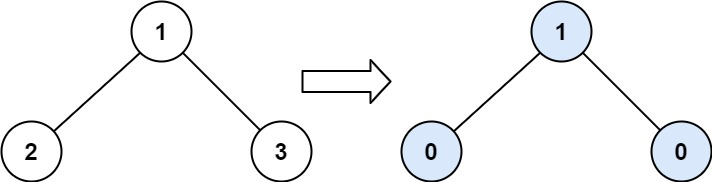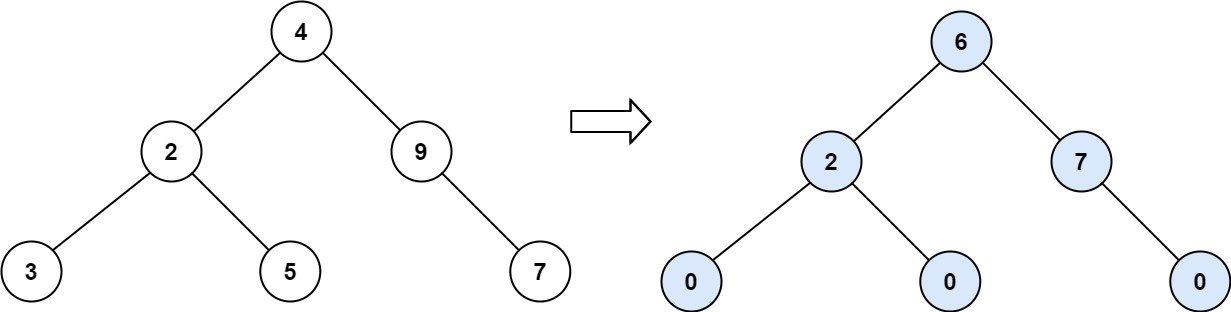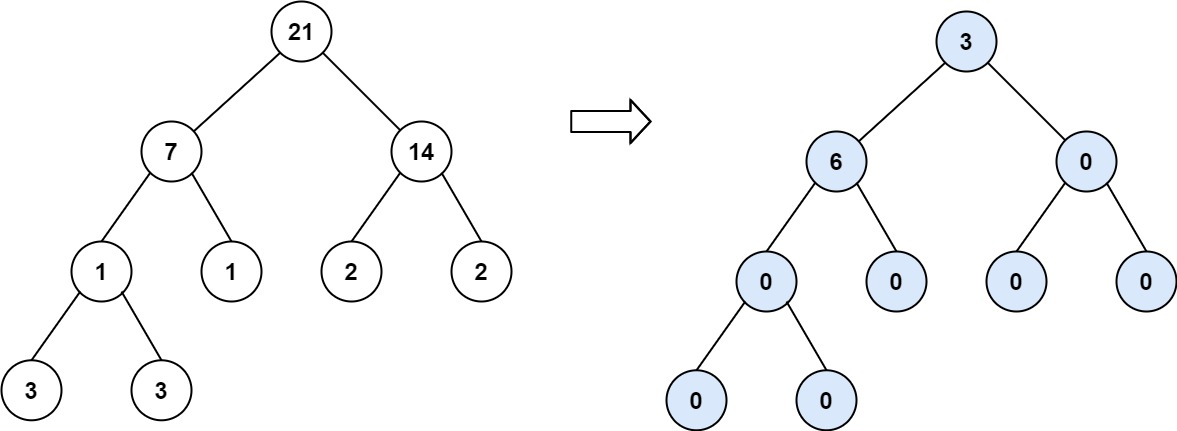563. Binary Tree Tilt

Given the `root` of a binary tree, return the sum of every tree node's tilt.

The tilt of a tree node is the absolute difference between the sum of all left subtree node values and all right subtree node values. If a node does not have a left child, then the sum of the left subtree node values is treated as `0`. The rule is similar if there the node does not have a right child.

Example 1:```Input: root = [1,2,3]
Output: 1
Explanation:
Tilt of node 2 : |0-0| = 0 (no children)
Tilt of node 3 : |0-0| = 0 (no children)
Tile of node 1 : |2-3| = 1 (left subtree is just left child, so sum is 2; right subtree is just right child, so sum is 3)
Sum of every tilt : 0 + 0 + 1 = 1
```

Example 2:```Input: root = [4,2,9,3,5,null,7]
Output: 15
Explanation:
Tilt of node 3 : |0-0| = 0 (no children)
Tilt of node 5 : |0-0| = 0 (no children)
Tilt of node 7 : |0-0| = 0 (no children)
Tilt of node 2 : |3-5| = 2 (left subtree is just left child, so sum is 3; right subtree is just right child, so sum is 5)
Tilt of node 9 : |0-7| = 7 (no left child, so sum is 0; right subtree is just right child, so sum is 7)
Tilt of node 4 : |(3+5+2)-(9+7)| = |10-16| = 6 (left subtree values are 3, 5, and 2, which sums to 10; right subtree values are 9 and 7, which sums to 16)
Sum of every tilt : 0 + 0 + 0 + 2 + 7 + 6 = 15
```

Example 3:```Input: root = [21,7,14,1,1,2,2,3,3]
Output: 9
```

Constraints:

• The number of nodes in the tree is in the range `[0, 104]`.
• `-1000 <= Node.val <= 1000`

563. Binary Tree Tilt
``````struct Solution;
use rustgym_util::*;

trait Tilt {
fn find_tilt(&self, tilt: &mut i32) -> i32;
}

fn find_tilt(&self, tilt: &mut i32) -> i32 {
if let Some(node) = self {
let node = node.borrow();
let left = &node.left;
let right = &node.right;
let left_sum = left.find_tilt(tilt);
let right_sum = right.find_tilt(tilt);
*tilt += (left_sum - right_sum).abs();
node.val + left_sum + right_sum
} else {
0
}
}
}

impl Solution {
fn find_tilt(root: TreeLink) -> i32 {
let mut tilt = 0;
root.find_tilt(&mut tilt);
tilt
}
}

#[test]
fn test() {
let root = tree!(1, tree!(2), tree!(3));
assert_eq!(Solution::find_tilt(root), 1);
}
``````